Home > A2C > Chapter 9 > Lesson 9.2.2 > Problem9-95

9-95.
1. Consider the graph below. Homework Help ✎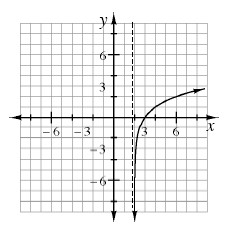1. What is the parent for this function?

2. What is the equation of the vertical asymptote?

3. Write a possible equation for this graph.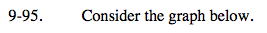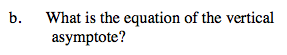At what point does the vertical asymptote cross the x-axis?

x = 2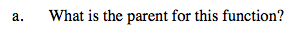Notice that it has a vertical asymptote.

y = log (x)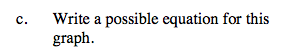How is the graph shifted?

y = log2(x − 2)